Start typing, then use the up and down arrows to select an option from the list.Jules Bruno
197views
4
Hey guys, in this new video, we're going to continue with our calculations off weak species. So in this question, it says an unknown weak base has an initial concentration off 0.750 Moeller, with a pH of 8.3 calculate its equilibrium based constant. So first of all, I say weak base. So that means ice chart. And then I want you to calculate the equilibrium base constant. So that means we have to find out what K B is. So what? The first thing we're gonna do is let's just write out the equation dealing with this week base. We can say here the week based could be represented by a minus if we want, and it's gonna react with water. Since it's a base, it's going to accept an H positive from the water and become h A water loses an h positive. So it's gonna become o H minus. Remember, Isis initial change equilibrium. We're gonna say water is a liquid. So in our ice chart, we put a big X through it because it doesn't matter Now we're gonna plug in the initial concentration of my week base. So here is gonna be 0.750 Moeller, I don't tell us anything initially about the products, so there's zero Don't And remember, we lose reactant to make products, so it's gonna be minus X plus X plus x 750 minus X plus X plus X. Now remember, K B equals products overreacting. So it's equal to H A times O H minus over a minus. What we're gonna do here is we can't ignore anything because we're looking for the value of K. So we're gonna plug in everything we know at equilibrium. So this is gonna be X times X. So this is gonna be X squared over 0. minus X. So in order to find K B, we need to know what the heck is X Now? I gave you a good piece of information here. I tell you what the Ph is, but remember what's wrong with this? PH goes with acids, but here we're dealing with the base. So we're gonna need P o. H. So what we're gonna do first is we're going to change the pH I give to you to P o. H. So we're gonna say P o h equals 14 minus p o p h. So that's gonna give me 5.97 And remember, if you know p o h u kno o h minus because o h minus equals 10 to the negative pH Yeah, and we're gonna say if we know the concentration of O H minus. What is this number fit into our calculations? Well, if you know h minus is calm concentration. You know what O H minus is at equilibrium and at equilibrium. Oh, h minus equals X. So this answer right? He will give us our variable for X R amount for X, find that X, Plug it into here, Plug it into here and we'll know what r k B is. So we're gonna say this is 1.7 times 10 to the negative six, and this equals R X. So take that number, plug it in. So I'm gonna have 1.7 times 10 to the minus six squared, divided by 0.750 minus 107 times 10 to the negative six. When we work all that out that I'll give us our KB value and r K B value will be 1.53 times 10 to the negative 12. That will be arcade value now. This ice chart was definitely different than the other one we did, but it's still dealing with the week species, so we have to use a nice chart. Now that we've seen this example, I want you guys to attempt to do the practice one on the bottom. So here the practice question says, determine the pH of a solution made by dissolving 6.1 g off sodium cyanide and a CNN in enough water to make 500 MLS of solution. The K A of H C N is 4.9 times 10 to the negative. 10. I'll give you guys a huge hint. We're dealing with this compound here. Determine what that compound is. Is it a base, or is it an acid? Is it weak or strong? That will help guide us on what to do if we know it's a week Species. We have to do a nice chart and remember, if it's a weak acid, then it uses K A. If it's a week base, then it uses K B. And remember, if you have to convert K to something else. Remember, the equation is K w equals K a times K B. So from those hints, use that to help you solve the question. And remember, when it comes to a nice chart, the units have to be in polarity, moles over leaders. The luck on this one, guys.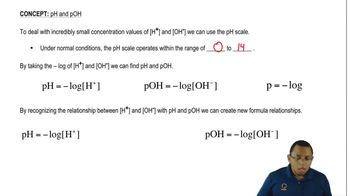01:31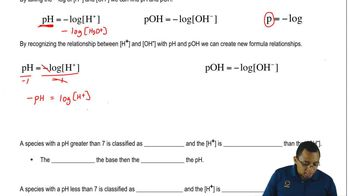01:35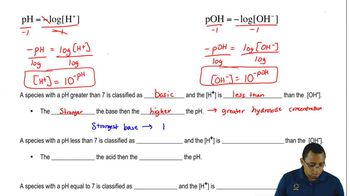02:42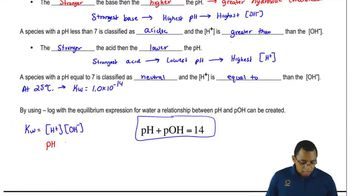01:21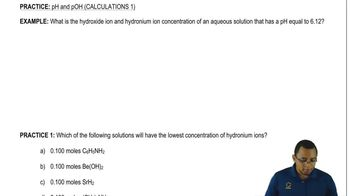03:15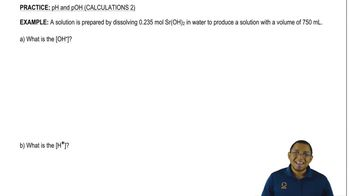04:07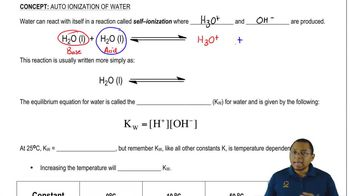03:10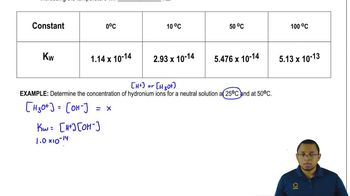02:47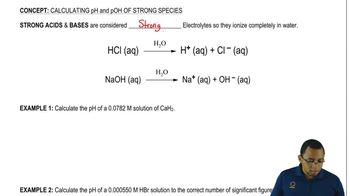01:32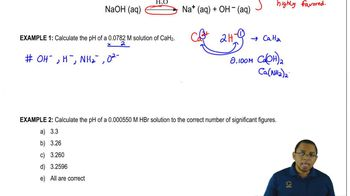04:48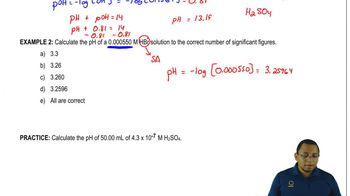03:29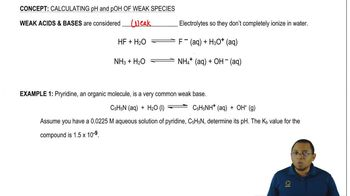00:57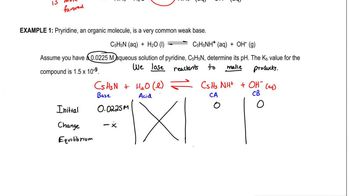09:40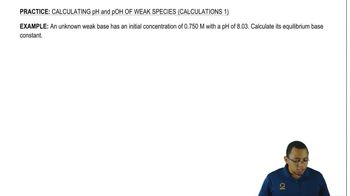05:31